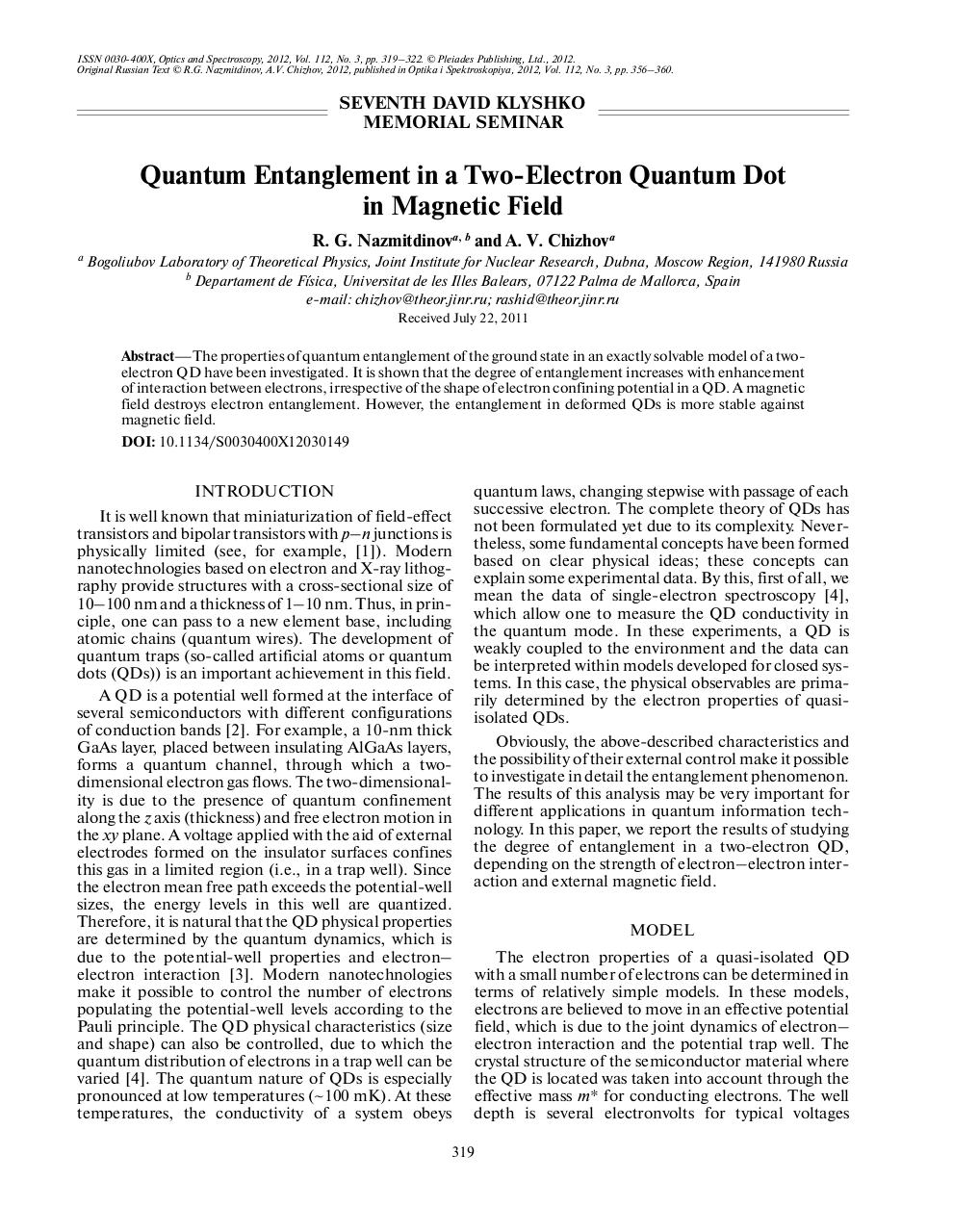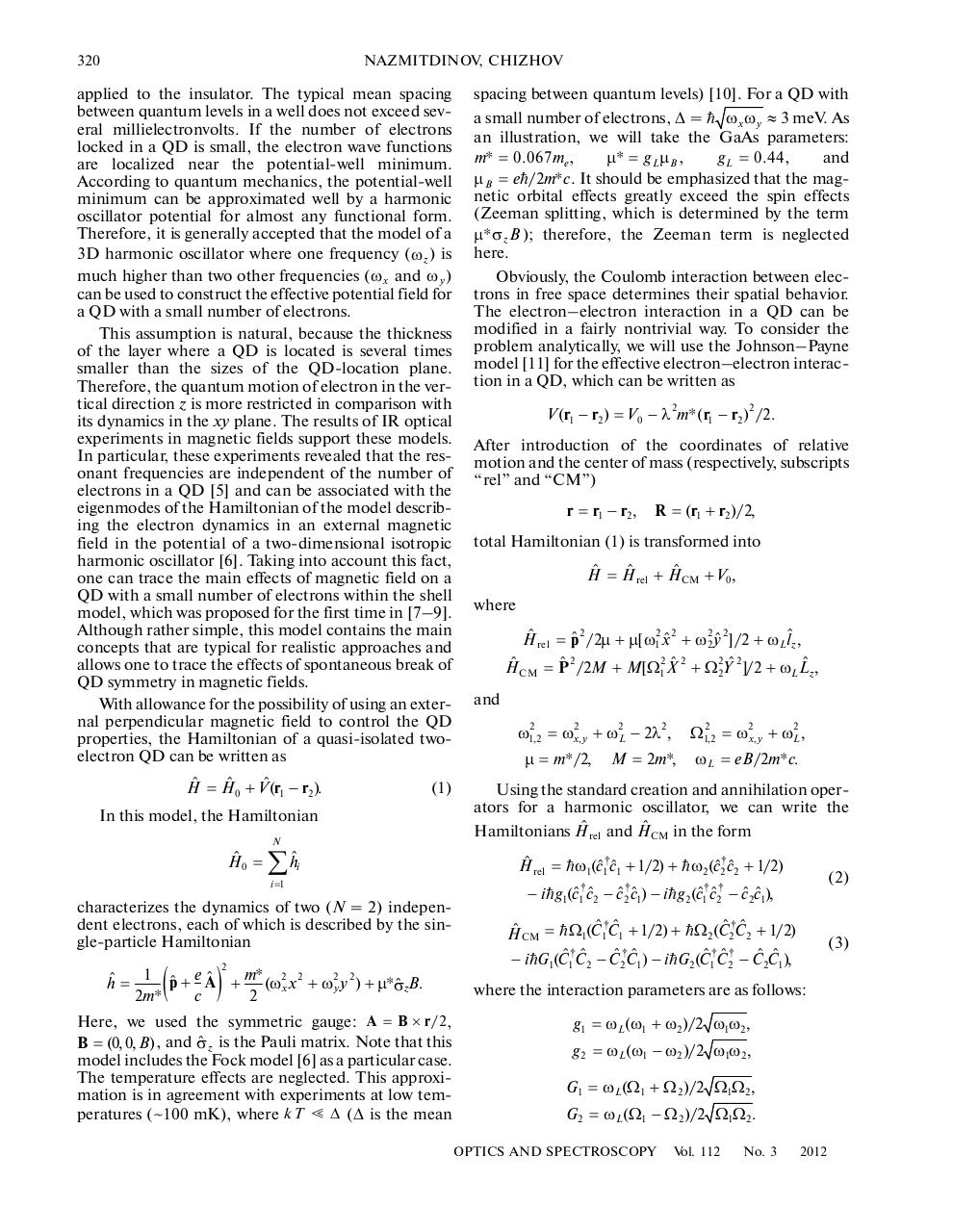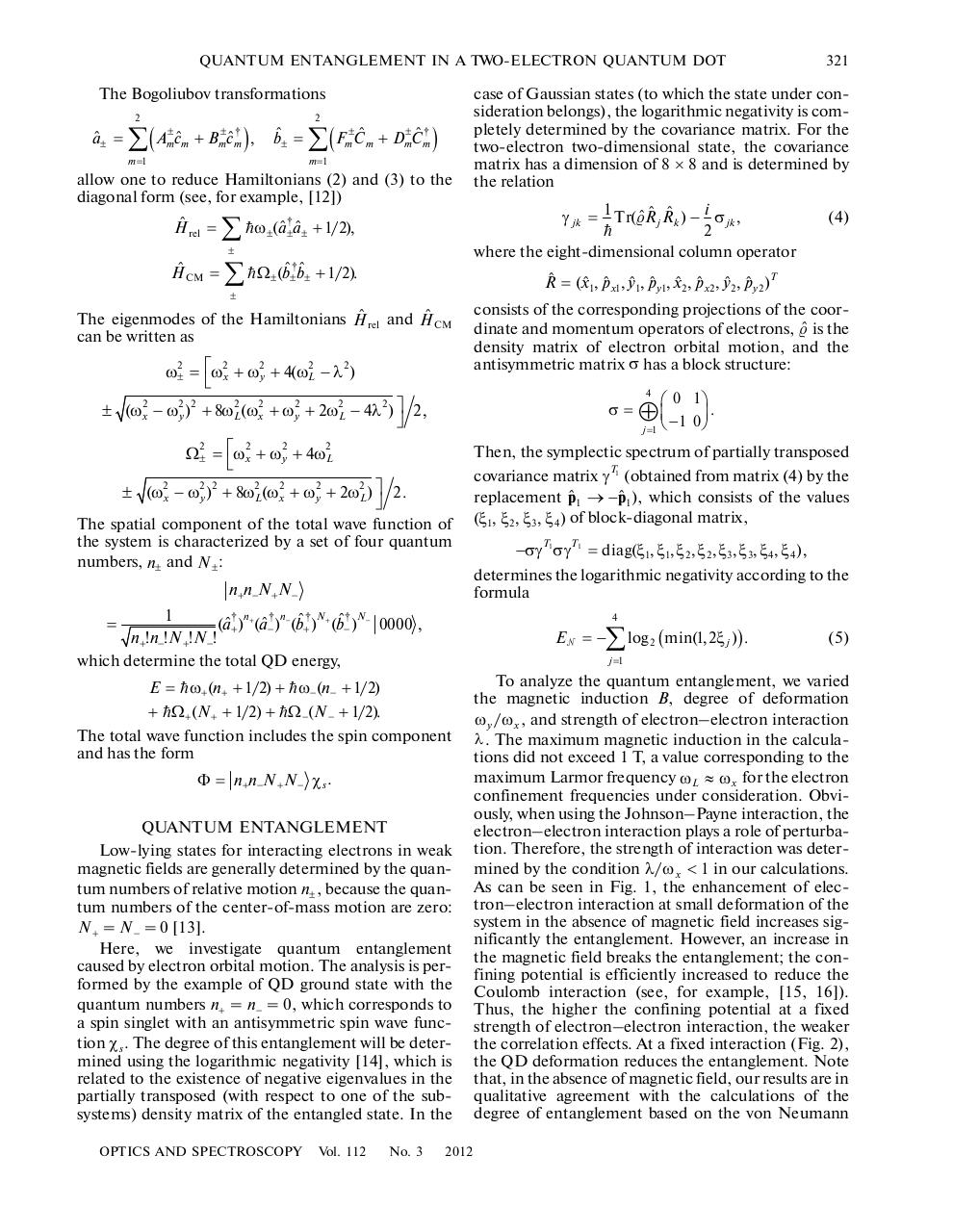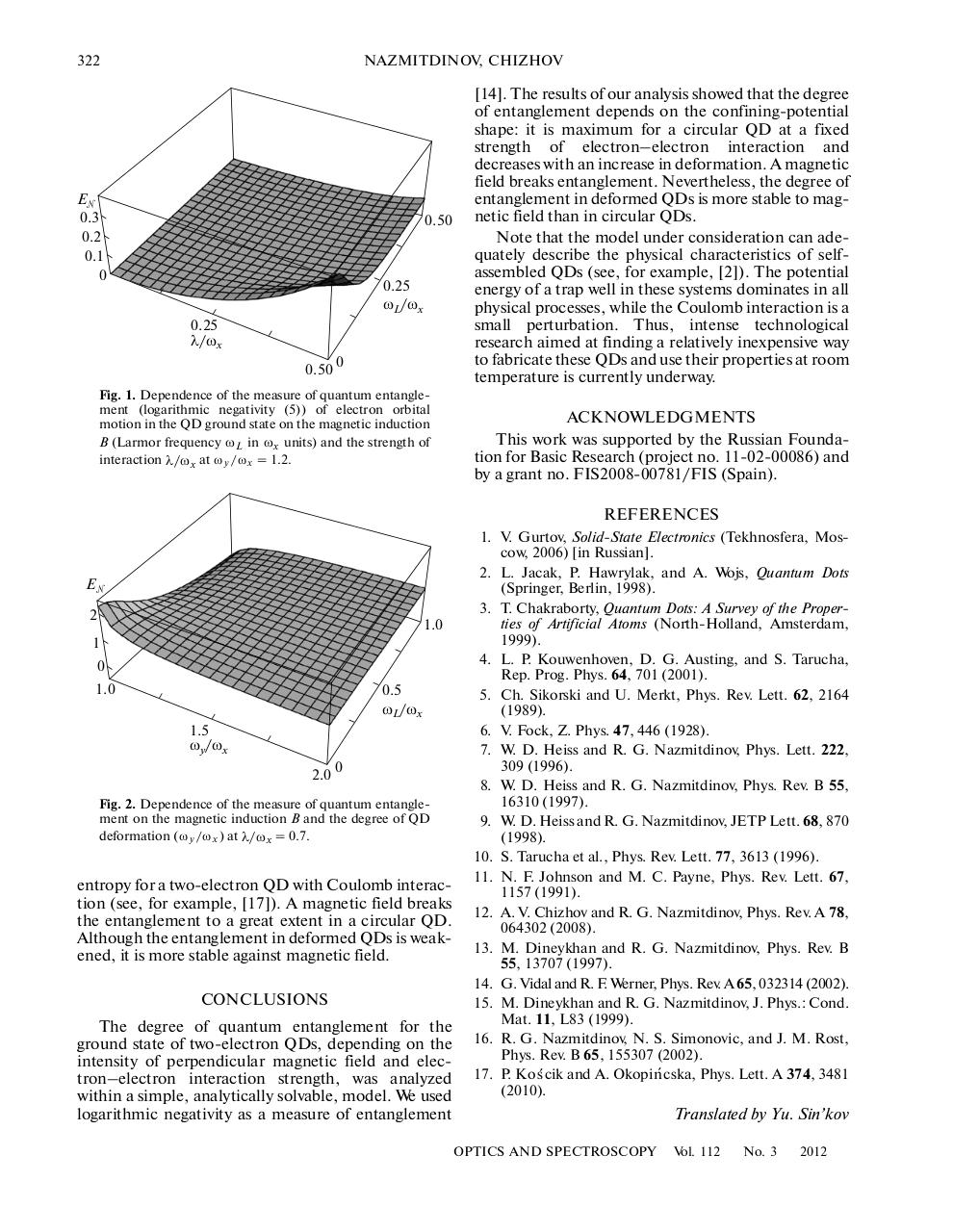# Quantum Entanglement Quantum Dot Magnetic Field .pdf

### File information

Original filename: Quantum Entanglement Quantum Dot Magnetic Field.pdf

This PDF 1.3 document has been generated by PScript5.dll Version 5.2.2 / Acrobat Distiller Server 8.1.0 (Sparc Solaris, Built: 2007-09-07), and has been sent on pdf-archive.com on 12/03/2016 at 21:33, from IP address 185.65.x.x. The current document download page has been viewed 531 times.
File size: 361 KB (4 pages).
Privacy: public file

Quantum Entanglement Quantum Dot Magnetic Field.pdf (PDF, 361 KB)

### Document preview

ISSN 0030400X, Optics and Spectroscopy, 2012, Vol. 112, No. 3, pp. 319–322. © Pleiades Publishing, Ltd., 2012.
Original Russian Text © R.G. Nazmitdinov, A.V. Chizhov, 2012, published in Optika i Spektroskopiya, 2012, Vol. 112, No. 3, pp. 356–360.

SEVENTH DAVID KLYSHKO
MEMORIAL SEMINAR

Quantum Entanglement in a TwoElectron Quantum Dot
in Magnetic Field
R. G. Nazmitdinova, b and A. V. Chizhova
a

Bogoliubov Laboratory of Theoretical Physics, Joint Institute for Nuclear Research, Dubna, Moscow Region, 141980 Russia
b
Departament de Física, Universitat de les Illes Balears, 07122 Palma de Mallorca, Spain
email: chizhov@theor.jinr.ru; rashid@theor.jinr.ru

Abstract—The properties of quantum entanglement of the ground state in an exactly solvable model of a two
electron QD have been investigated. It is shown that the degree of entanglement increases with enhancement
of interaction between electrons, irrespective of the shape of electron confining potential in a QD. A magnetic
field destroys electron entanglement. However, the entanglement in deformed QDs is more stable against
magnetic field.
DOI: 10.1134/S0030400X12030149

INTRODUCTION
It is well known that miniaturization of fieldeffect
transistors and bipolar transistors with p–n junctions is
physically limited (see, for example, ). Modern
nanotechnologies based on electron and Xray lithog
raphy provide structures with a crosssectional size of
10–100 nm and a thickness of 1–10 nm. Thus, in prin
ciple, one can pass to a new element base, including
atomic chains (quantum wires). The development of
quantum traps (socalled artificial atoms or quantum
dots (QDs)) is an important achievement in this field.
A QD is a potential well formed at the interface of
several semiconductors with different configurations
of conduction bands . For example, a 10nm thick
GaAs layer, placed between insulating AlGaAs layers,
forms a quantum channel, through which a two
dimensional electron gas flows. The twodimensional
ity is due to the presence of quantum confinement
along the z axis (thickness) and free electron motion in
the xy plane. A voltage applied with the aid of external
electrodes formed on the insulator surfaces confines
this gas in a limited region (i.e., in a trap well). Since
the electron mean free path exceeds the potentialwell
sizes, the energy levels in this well are quantized.
Therefore, it is natural that the QD physical properties
are determined by the quantum dynamics, which is
due to the potentialwell properties and electron–
electron interaction . Modern nanotechnologies
make it possible to control the number of electrons
populating the potentialwell levels according to the
Pauli principle. The QD physical characteristics (size
and shape) can also be controlled, due to which the
quantum distribution of electrons in a trap well can be
varied . The quantum nature of QDs is especially
pronounced at low temperatures (~100 mK). At these
temperatures, the conductivity of a system obeys

quantum laws, changing stepwise with passage of each
successive electron. The complete theory of QDs has
not been formulated yet due to its complexity. Never
theless, some fundamental concepts have been formed
based on clear physical ideas; these concepts can
explain some experimental data. By this, first of all, we
mean the data of singleelectron spectroscopy ,
which allow one to measure the QD conductivity in
the quantum mode. In these experiments, a QD is
weakly coupled to the environment and the data can
be interpreted within models developed for closed sys
tems. In this case, the physical observables are prima
rily determined by the electron properties of quasi
isolated QDs.
Obviously, the abovedescribed characteristics and
the possibility of their external control make it possible
to investigate in detail the entanglement phenomenon.
The results of this analysis may be very important for
different applications in quantum information tech
nology. In this paper, we report the results of studying
the degree of entanglement in a twoelectron QD,
depending on the strength of electron–electron inter
action and external magnetic field.
MODEL
The electron properties of a quasiisolated QD
with a small number of electrons can be determined in
terms of relatively simple models. In these models,
electrons are believed to move in an effective potential
field, which is due to the joint dynamics of electron–
electron interaction and the potential trap well. The
crystal structure of the semiconductor material where
the QD is located was taken into account through the
effective mass m* for conducting electrons. The well
depth is several electronvolts for typical voltages

319

320

NAZMITDINOV, CHIZHOV

applied to the insulator. The typical mean spacing
between quantum levels in a well does not exceed sev
eral millielectronvolts. If the number of electrons
locked in a QD is small, the electron wave functions
are localized near the potentialwell minimum.
According to quantum mechanics, the potentialwell
minimum can be approximated well by a harmonic
oscillator potential for almost any functional form.
Therefore, it is generally accepted that the model of a
3D harmonic oscillator where one frequency (ω z ) is
much higher than two other frequencies (ω x and ω y )
can be used to construct the effective potential field for
a QD with a small number of electrons.
This assumption is natural, because the thickness
of the layer where a QD is located is several times
smaller than the sizes of the QDlocation plane.
Therefore, the quantum motion of electron in the ver
tical direction z is more restricted in comparison with
its dynamics in the xy plane. The results of IR optical
experiments in magnetic fields support these models.
In particular, these experiments revealed that the res
onant frequencies are independent of the number of
electrons in a QD  and can be associated with the
eigenmodes of the Hamiltonian of the model describ
ing the electron dynamics in an external magnetic
field in the potential of a twodimensional isotropic
harmonic oscillator . Taking into account this fact,
one can trace the main effects of magnetic field on a
QD with a small number of electrons within the shell
model, which was proposed for the first time in [7–9].
Although rather simple, this model contains the main
concepts that are typical for realistic approaches and
allows one to trace the effects of spontaneous break of
QD symmetry in magnetic fields.
With allowance for the possibility of using an exter
nal perpendicular magnetic field to control the QD
properties, the Hamiltonian of a quasiisolated two
electron QD can be written as

Hˆ = Hˆ 0 + Vˆ(r1 − r2 ).

(1)

In this model, the Hamiltonian
N

Hˆ 0 =

∑ hˆ

i

i =1

characterizes the dynamics of two (N = 2) indepen
dent electrons, each of which is described by the sin
gleparticle Hamiltonian

(

)

2

ˆ + m* (ω2x x 2 + ω2y y 2 ) + μ*σ
hˆ = 1 pˆ + e A
ˆ zB.
c
2m*
2
Here, we used the symmetric gauge: A = B × r /2 ,
ˆ z is the Pauli matrix. Note that this
B = (0, 0, B) , and σ
model includes the Fock model  as a particular case.
The temperature effects are neglected. This approxi
mation is in agreement with experiments at low tem
peratures (~100 mK), where kT Ⰶ Δ (Δ is the mean

spacing between quantum levels) . For a QD with
a small number of electrons, Δ =  ω x ω y ≈ 3 meV. As
an illustration, we will take the GaAs parameters:
and
m* = 0 . 067me,
µ* = g Lµ B ,
g L = 0 . 44 ,
µ B = e/2m*c . It should be emphasized that the mag
netic orbital effects greatly exceed the spin effects
(Zeeman splitting, which is determined by the term
μ* σ z B ); therefore, the Zeeman term is neglected
here.
Obviously, the Coulomb interaction between elec
trons in free space determines their spatial behavior.
The electron–electron interaction in a QD can be
modified in a fairly nontrivial way. To consider the
problem analytically, we will use the Johnson–Payne
model  for the effective electron–electron interac
tion in a QD, which can be written as

V (r1 − r2 ) = V0 − λ 2m*( r1 − r2 )2 /2.
After introduction of the coordinates of relative
motion and the center of mass (respectively, subscripts
“rel” and “CM”)

r = r1 − r2,

R = (r1 + r2 )/2,

total Hamiltonian (1) is transformed into

Hˆ = Hˆ rel + Hˆ CM + V0,
where
2
2 2
2 2
Hˆ rel = pˆ /2μ + μ[ ω1 xˆ + ω2 yˆ ]/2 + ωLlˆz ,
ˆ 2 /2M + M[Ω12 Xˆ 2 + Ω 22Yˆ 2]/2 + ωL Lˆz ,
Hˆ CM = P

and

ω12,2 = ω2x, y + ω2L − 2λ 2, Ω12,2 = ω2x, y + ω2L,
μ = m*/2, M = 2m*, ωL = eB /2m*c.
Using the standard creation and annihilation oper
ators for a harmonic oscillator, we can write the
Hamiltonians Hˆ rel and Hˆ CM in the form

Hˆ rel = ω1(cˆ1†cˆ1 + 1/2) + ω2(cˆ2†cˆ2 + 1/2)

(2)

− i g1(cˆ1†cˆ2 − cˆ2†cˆ1) − i g 2(cˆ1†cˆ2† − cˆ2cˆ1),

Hˆ CM =  Ω1(Cˆ1 Cˆ1 + 1/2) +  Ω 2(Cˆ2 Cˆ2 + 1/2)

† †
− i  G1(Cˆ1 Cˆ2 − Cˆ2 Cˆ1) − i G2(Cˆ1 Cˆ2 − Cˆ2Cˆ1),

(3)

where the interaction parameters are as follows:

g1 = ωL(ω1 + ω2 )/2 ω1ω2,
g 2 = ωL(ω1 − ω2 )/2 ω1ω2,
G1 = ωL(Ω1 + Ω 2 )/2 Ω1Ω 2,
G 2 = ωL(Ω1 − Ω 2 )/2 Ω1Ω 2.
OPTICS AND SPECTROSCOPY

Vol. 112

No. 3

2012

QUANTUM ENTANGLEMENT IN A TWOELECTRON QUANTUM DOT

The Bogoliubov transformations
2

aˆ± =

∑(

Am±cˆm

+

Bm±cˆm†

m =1

2

),

bˆ± =

∑(F

±ˆ
m Cm

+ Dm±Cˆm†

m =1

)

allow one to reduce Hamiltonians (2) and (3) to the
diagonal form (see, for example, )

Hˆ rel =

ω±(aˆ±†aˆ± + 1/2),

±

Hˆ CM =

∑ Ω (bˆ bˆ
±

± ±

+ 1/2).

The eigenmodes of the Hamiltonians Hˆ rel and Hˆ CM
can be written as

ω± = ⎡ωx + ωy + 4(ωL − λ )

2

2

2

case of Gaussian states (to which the state under con
sideration belongs), the logarithmic negativity is com
pletely determined by the covariance matrix. For the
twoelectron twodimensional state, the covariance
matrix has a dimension of 8 × 8 and is determined by
the relation

γ jk = 1 Tr(ˆRˆ j Rˆk ) − i σ jk ,

2
where the eightdimensional column operator

2

consists of the corresponding projections of the coor
dinate and momentum operators of electrons, ˆ is the
density matrix of electron orbital motion, and the
antisymmetric matrix σ has a block structure:
4

± (ω2x − ω2y )2 + 8ω2L(ω2x + ω2y + 2ω2L − 4λ 2 ) ⎤ 2 ,

2
Ω±

=

⎡ω2x

+

2
ωy

+

σ=

n+n−N +N −
1
(aˆ+†)n+ (aˆ−†)n− (bˆ+†)N + (bˆ−†)N − 0000 ,
n+!n−!N +!N −!
which determine the total QD energy,
=

−σγ T1σγ T1 = diag(ξ1, ξ1, ξ 2, ξ 2, ξ3, ξ3, ξ 4 , ξ 4 ) ,
determines the logarithmic negativity according to the
formula

∑ log

Eᏺ = −

2

( min(1, 2ξ j )) .

(5)

j =1

Φ = n+n−N + N − χ s .
QUANTUM ENTANGLEMENT
Lowlying states for interacting electrons in weak
magnetic fields are generally determined by the quan
tum numbers of relative motion n± , because the quan
tum numbers of the centerofmass motion are zero:
N + = N − = 0 .
Here, we investigate quantum entanglement
caused by electron orbital motion. The analysis is per
formed by the example of QD ground state with the
quantum numbers n+ = n− = 0, which corresponds to
a spin singlet with an antisymmetric spin wave func
tion χ s . The degree of this entanglement will be deter
mined using the logarithmic negativity , which is
related to the existence of negative eigenvalues in the
partially transposed (with respect to one of the sub
systems) density matrix of the entangled state. In the
No. 3

Then, the symplectic spectrum of partially transposed
covariance matrix γT1 (obtained from matrix (4) by the
replacement pˆ 1 → −pˆ 1), which consists of the values
(ξ1, ξ 2, ξ3, ξ 4 ) of blockdiagonal matrix,

4

E = ω+(n+ + 1/2) + ω−(n− + 1/2)
+ Ω +(N + + 1/2) + Ω −(N − + 1/2).
The total wave function includes the spin component
and has the form

Vol. 112

⎛ 0 1⎞

⊕ ⎜⎝ −1 0 ⎟⎠ .
j =1

4ω2L

± (ω2x − ω2y )2 + 8ω2L(ω2x + ω2y + 2ω2L) ⎤ 2 .

The spatial component of the total wave function of
the system is characterized by a set of four quantum
numbers, n± and N ± :

OPTICS AND SPECTROSCOPY

(4)

Rˆ = (xˆ1, pˆ x1, yˆ1, pˆ y1, xˆ2, pˆ x2, yˆ2, pˆ y2 )T

±

2

321

2012

To analyze the quantum entanglement, we varied
the magnetic induction B, degree of deformation
ω y / ω x , and strength of electron–electron interaction
λ . The maximum magnetic induction in the calcula
tions did not exceed 1 T, a value corresponding to the
maximum Larmor frequency ωL ≈ ω x for the electron
confinement frequencies under consideration. Obvi
ously, when using the Johnson–Payne interaction, the
electron–electron interaction plays a role of perturba
tion. Therefore, the strength of interaction was deter
mined by the condition λ / ω x &lt; 1 in our calculations.
As can be seen in Fig. 1, the enhancement of elec
tron–electron interaction at small deformation of the
system in the absence of magnetic field increases sig
nificantly the entanglement. However, an increase in
the magnetic field breaks the entanglement; the con
fining potential is efficiently increased to reduce the
Coulomb interaction (see, for example, [15, 16]).
Thus, the higher the confining potential at a fixed
strength of electron–electron interaction, the weaker
the correlation effects. At a fixed interaction (Fig. 2),
the QD deformation reduces the entanglement. Note
that, in the absence of magnetic field, our results are in
qualitative agreement with the calculations of the
degree of entanglement based on the von Neumann

322

NAZMITDINOV, CHIZHOV

Eᏺ
0.3
0.2
0.1
0

0.50

0.25
ωL/ωx
0.25
λ/ωx
0.50 0

Fig. 1. Dependence of the measure of quantum entangle
ment (logarithmic negativity (5)) of electron orbital
motion in the QD ground state on the magnetic induction
B (Larmor frequency ω L in ω x units) and the strength of
interaction λ/ ω x at ω y / ω x = 1.2.

. The results of our analysis showed that the degree
of entanglement depends on the confiningpotential
shape: it is maximum for a circular QD at a fixed
strength of electron–electron interaction and
decreases with an increase in deformation. A magnetic
field breaks entanglement. Nevertheless, the degree of
entanglement in deformed QDs is more stable to mag
netic field than in circular QDs.
Note that the model under consideration can ade
quately describe the physical characteristics of self
assembled QDs (see, for example, ). The potential
energy of a trap well in these systems dominates in all
physical processes, while the Coulomb interaction is a
small perturbation. Thus, intense technological
research aimed at finding a relatively inexpensive way
to fabricate these QDs and use their properties at room
temperature is currently underway.
ACKNOWLEDGMENTS
This work was supported by the Russian Founda
tion for Basic Research (project no. 110200086) and
by a grant no. FIS200800781/FIS (Spain).
REFERENCES

Eᏺ
2

1.0

1
0
1.0

0.5
ωL/ωx
1.5
ωy/ωx
2.0

0

Fig. 2. Dependence of the measure of quantum entangle
ment on the magnetic induction B and the degree of QD
deformation (ω y / ω x ) at λ/ ω x = 0.7.

entropy for a twoelectron QD with Coulomb interac
tion (see, for example, ). A magnetic field breaks
the entanglement to a great extent in a circular QD.
Although the entanglement in deformed QDs is weak
ened, it is more stable against magnetic field.
CONCLUSIONS
The degree of quantum entanglement for the
ground state of twoelectron QDs, depending on the
intensity of perpendicular magnetic field and elec
tron–electron interaction strength, was analyzed
within a simple, analytically solvable, model. We used
logarithmic negativity as a measure of entanglement

1. V. Gurtov, SolidState Electronics (Tekhnosfera, Mos
cow, 2006) [in Russian].
2. L. Jacak, P. Hawrylak, and A. Wojs, Quantum Dots
(Springer, Berlin, 1998).
3. T. Chakraborty, Quantum Dots: A Survey of the Proper
ties of Artificial Atoms (NorthHolland, Amsterdam,
1999).
4. L. P. Kouwenhoven, D. G. Austing, and S. Tarucha,
Rep. Prog. Phys. 64, 701 (2001).
5. Ch. Sikorski and U. Merkt, Phys. Rev. Lett. 62, 2164
(1989).
6. V. Fock, Z. Phys. 47, 446 (1928).
7. W. D. Heiss and R. G. Nazmitdinov, Phys. Lett. 222,
309 (1996).
8. W. D. Heiss and R. G. Nazmitdinov, Phys. Rev. B 55,
16310 (1997).
9. W. D. Heiss and R. G. Nazmitdinov, JETP Lett. 68, 870
(1998).
10. S. Tarucha et al., Phys. Rev. Lett. 77, 3613 (1996).
11. N. F. Johnson and M. C. Payne, Phys. Rev. Lett. 67,
1157 (1991).
12. A. V. Chizhov and R. G. Nazmitdinov, Phys. Rev. A 78,
064302 (2008).
13. M. Dineykhan and R. G. Nazmitdinov, Phys. Rev. B
55, 13707 (1997).
14. G. Vidal and R. F. Werner, Phys. Rev. A 65, 032314 (2002).
15. M. Dineykhan and R. G. Nazmitdinov, J. Phys.: Cond.
Mat. 11, L83 (1999).
16. R. G. Nazmitdinov, N. S. Simonovic, and J. M. Rost,
Phys. Rev. B 65, 155307 (2002).
17. P. Ko ´s cik and A. Okopi n´ cska, Phys. Lett. A 374, 3481
(2010).

Translated by Yu. Sin’kov
OPTICS AND SPECTROSCOPY

Vol. 112

No. 3

2012#### HTML Code

Copy the following HTML code to share your document on a Website or Blog

#### QR Code### Related keywords